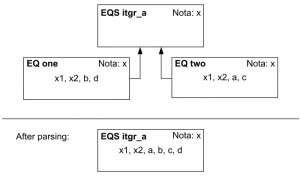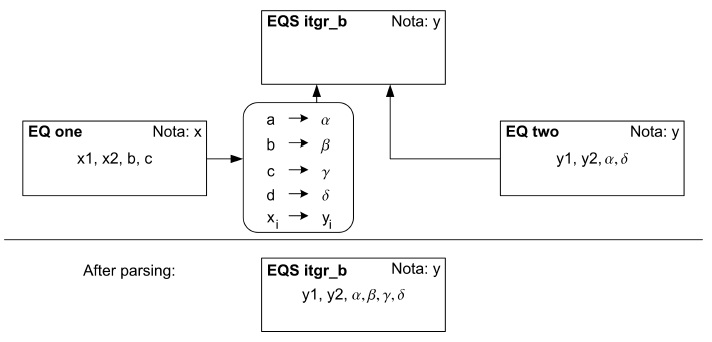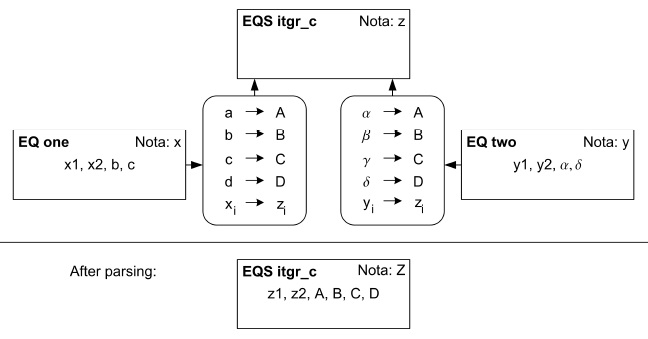# Connection Techniques I – Naming Policy ‘integrate’

Stefan Kuntsche, Sanam Sabeti Adibpour, Gregor Tolksdorf

This tutorial section covers the basic use of equations or equation systems that use different notations. It is assumed that you have worked through the basic tutorial parts.

Rules

The basic contract of the naming policy ‘integrate’ is as follows:

• Basic rule: If an equation or equation system (sub element) is added to a super equation system (super element), the sub element’s variables will get the namespace of the super element
• Additional rule using different notations: If a sub element is connected to a super element, and both elements use different notations, then all variable names of the sub element have to be translated (by a connector) into the super notation.
• Attention: In any time the meaning of each variable has to be uniquely defined by the notation of its namespace. If different notations are used in sub and super element, each variable has to be translated. Otherwise the meaning of some variables coming from the sub element would be undefined in the notation (and therefore in the namespace ) of the super element.
• Result: After parsing, all variables have a top level naming according to the super notation in the top level namespace.

‘Integrate’ case a: All elements use the same notation.

This is the standard case where no translation is necessary. It is only listed here for the sake of completeness.Figure 1: Graph of the variable interpretation

Equation System itgr a
$latex x_{i=1} + b\cdot x_{i=2} = c$
$latex d\cdot (x_{i=2})^{2} = -a + x_{i=1}$

Notation x

Base Names:

[table sort=”desc,asc”]
Name,Description
$latex x$,value x
$latex a$,parameter a
$latex b$,parameter b
$latex c$,parameter c
$latex d$,parameter d
[/table]

Indices:

[table sort=”desc,asc”]
Name,max val,Description
$latex i$,Ni,value index
[/table]

Problem description

Design variables and their corresponding values:

$latex a = 1$

$latex b = 3$

$latex c = -0.5$

$latex d = 1$

Iteration variables and their corresponding guess values:

$latex x_{i=1} = 5$

$latex x_{i=2} = 5$

Expected solution:

$latex x_{i=1} = -17$

$latex x_{i=2} = 6$

Repeating the basics If you do not know how to enter the above equation system and solve the derived problem in MOSAICmodeling, then you should work through (or revisit) the tutorial parts in the basics.

‘Integrate’ case b: A connected equation uses another notation.

In this case (only) one sub equation has a different notation. This is similar to the scenario where you establish your equations in your notation and use some equations from a collaborating developer who uses his own notation.

Equation System itgr b

$latex y_{j=1} + \beta \cdot y_{j=2} = \gamma$

$latex \delta \cdot (y_{j=2})^{2} = -\alpha + y_{j=1}$

Notation y

Base Names:

[table sort=”desc,asc”]
Name,Description
$latex y$,values
$latex \alpha$,parameter 1
$latex \beta$,parameter 2
$latex \gamma$,parameter 3
$latex \delta$,parameter 4
[/table]

Indices:

[table sort=”desc,asc”]
Name,Max Val,Description
$latex j$,Nj,value index
[/table]

Collaboration

Equation (\ref{eq:itgr:b:incl}) exists in a collaborating project. To avoid reimplementation the existing equation (\ref{eq:itgr:b:used}) is used here. In the other project another notation is used. To be able to use equations of the other project, a connector must be created, that translates into the notation used in this project.

Equation one

$latex x_{i=1} + b \cdot x_{i=2} = c$

Notation x

Base Names:

[table sort=”desc,asc”]
Name,Description
$latex x$,values
$latex a$, parameter 1
$latex b$,parameter 2
$latex c$,parameter3
$latex d$,parameter 4
[/table]

Indices:

[table sort=”desc,asc”]
Name,Max val,Description
$latex i$,Ni,value index
[/table]

Connector one

• Subnotation: Notation x
• Supernotation: Notation y
• Value list:

$latex x_{i} \rightarrow y_{j}$
$latex a \rightarrow \alpha$
$latex b \rightarrow \beta$
$latex c \rightarrow \gamma$
$latex d \rightarrow \delta$Figure 2: Graph of the variable interpretation

Problem description

Design variables with their values:

$latex \alpha = 2$

$latex \beta = 3$

$latex \delta = 2$

$latex \gamma = 3$

Iteration variables with guess values:

$latex y_{j=1} = 1$

$latex y_{j=2} = 1$

Expected solution:

$latex y_{j=1} = 2.16$

$latex y_{j=2} = 0.28$

Creating the equations and the notations As a preparation of this tutorial the basic model elements must be created:

• Create ‘Notation y’ and ‘Equation two’ as it is used in your own project (see eq. \ref{eq:itgr:b:eq:two:new}).
• Create ‘Notation x’ and ‘Equation one’ as it is present in the collaborating project (see eq. \ref{eq:itgr:b:used}).

Creating the connector
Now it is necessary to create a connector, which is basically a list of synonymous variable names. The connector to be created is given in the specifications above but repeated here for convenience:

Connector one

• Subnotation: Notation x
• Supernotation: Notation y
• Value list:

$latex x_{i} \rightarrow y_{j}$
$latex a \rightarrow \alpha$
$latex b \rightarrow \beta$
$latex c \rightarrow \gamma$
$latex d \rightarrow \delta$

Every connector consists of three pieces of information: The sub notation, the super notation and a variable matching list. However, there are several ways to create a connector. The standard way is to specify the sub and super notation directly and create the variable namings that need to be matched by hand. If you already have connectible elements (equations or equation systems) that you want to match, you can also load them into the Connector Editor. In this case the notations and the variable namings are extracted from the loaded connected elements, which usually saves much work. The stored information, however, is still only the sub notation, the super notation and the variable matching list. In this introductory example the latter case is shown. To create the connector based on existing connectible elements do the following:

• Select the Connector tab in the Editor Bar.
• Activate the tab Set Notations. You see two sections, one for the Sub Notation and one for the Super Notation. Both sections contain a field to import the notation.
• In the section for the Sub Notation press the [Import] button of the associated file selection area.
• Select the file for ‘Equation one’.
• Let’s take a look at the results of the last action:
• The Notation file panel shows the notation used by ‘Equation one’.
• If you look at View section you will find the notation has been loaded into a detailed view panel similar to the Notation Editor with the title Sub Notation
• Go back to the tab Set Notations.
• Within the specification of the Super Element press the [Import] button on the right hand side of this area.
• Now select the file for ‘Equation two’. (Strictly, ‘itgr b’ is the super element.  But ‘Equation two’ uses the same notation and contains all necessary variables names).
• The variable names of ‘Equation two’ and information about the corresponding notation, ‘Notation y’ should be present in the editor now, just as it was shown for the sub element.
• Now the matching of the variables must be specified. Change to the tab Edit Matching.
• For the correct translation of the variables of ‘Equation one’ it is sufficient to specify a matching for bc, and $latex x_{i}$.
• We will start with b.  press [New], choose Sub variable Naming and type b into the field Tex Expression. Press [Update MathML] and then [OK].  To specify the corresponding name, $latex \beta$, press [New], choose Super variable Naming and type \beta into the field Tex Expression. Press [Update MathML] and then [OK].
• Select both b in the Sub Notation list and $latex \beta$ in the Super Notation list and press [Match].
• It is useful to test the translation results of the new connector. To do so, select the tab Test Connector and load the sub equation or equation system for which the translation should be tested. For this tutorial load ‘Equation one’ and look at the results.
• Add another synonym information for c, creating \gamma and matching the names as described in the previous step.
• Now you could match $latex x_{i=1}$ and $latex x_{i=2}$ separately to $latex y_{j=1}$ and $latex y_{j=2}$. However, this would not be very efficient. Instead you should match the indiced variable in a generic way:
• Create a New Sub $latex x_{i}$ and a New Super $latex y_{j}$ and match them.
• At all times you may look at the contents of the connector by selecting the tab View Connector. Further you may always look at the matching results in the tab Test Connector.
• Save the connector as ‘Connector one’.
• Up to now the connector is sufficient for the above example case. To obtain a connector that is able to translate all symbols of ‘Notation x’ into symbols of ‘Notation y’ you need to create the sub variable namings a and d and match them with the super variable namings $latex \alpha$ and $latex \delta$.

Using the connector (during creation of the equation system)

• Choose Equation System in the Editor Bar.
• First load ‘Notation y’ into the Notation section of this editor.
• To connect ‘Equation one’ press [Add].
• Select ‘Equation one’ as usual in the Edit Connection to Element but do not press [Submit].
• Choose the Naming Policy integrate.
• Still in the dialog check the box for Use connector.
• Press [Change] next to the now activated text field and choose the file for ‘Connector one’ that you created above.
• Press [Submit].
• The connector name is shown in the table row for ‘Equation one’.
• Add ‘Equation two’ in the usual way without specifying any connector.

Differences when evaluating the equation system

• In the Evaluate bar activate the tab Equation System and load the equation system as usual.
• You will see that the equations are presented in their original notation.
• Specify the maximum index value NC=2 in the Indexing tab.
• Now have a look at the presentation in the Instance tab. Here, both equations are  presented in the symbols corresponding to the super notation (‘Notation y’).
• Change to tab Variable Specification.
• Select the variable $latex \beta$. Remember that this variable has the variable name b in the original notation.
• Look at the lower end of the editor at the Notation Information Panel. At the left hand side you see the symbol $latex \beta$ and next to it an activated arrow button pointing down. Press this button [$latex \downarrow$]. You will see that the symbol changes from $latex \beta$ to b and the notation information at the right hand side shows the description for b provided by ‘Notation x’.
• Using the buttons [ $latex \uparrow$ ] and [ $latex \downarrow$ ] you can change between the two namings. Pressing [T] displays the Top Level Naming, which is the naming that is actually used to distinguish the variables from each other.
• Enter the variable specification given in the model and problem description and solve the problem.

‘Integrate’ case c: All connected equation systems use a different notation from the desired one.
This is the most general (but most rare) case. All connected sub elements use different notations from the super notation.

Equation System itgr c
$latex z_{k=1} + B \cdot z_{k=2} = C$

$latex D \cdot (z_{k=2})^{2} = -A + z_{k=1}$

Notation z
Base Names:

[table sort=”desc,asc”]
Name,Description
$latex z$,values
$latex A$,parameter 1
$latex B$,parameter 2
$latex C$,parameter 3
$latex D$,parameter 4
[/table]

Indices:

[table sort=”desc,asc”]
Name,Max val,Description
$latex k$,Nk,value index
[/table]

Collaboration

Equations (\ref{eq:itgr:c:incl:one}) and (\ref{eq:itgr:c:incl:two}) exist in other projects. To avoid reimplementation of the existing equations (\ref{eq:itgr:b:used}) and (\ref{eq:itgr:b:eq:two:new}) are used here. Applicable connectors are used to translate between the notations.

Equation one

$latex x_{i=1} + b \cdot x_{i=2} = c$

Notation x

Base Names:

[table sort=”desc,asc”]
Name,Description
$latex x$,values
$latex a$,parameter 1
$latex b$,parameter 2
$latex c$,parameter 3
$latex d$,parameter 4
[/table]

Indices:

[table sort=”desc,asc”]
Name,Max val,Description
i,Ni,value index
[/table]

Connector two

• Subnotation: Notation x
• Supernotation: Notation z
• Value list:

$latex x_{i}\rightarrow z_{k}$
$latex a\rightarrow A$
$latex b\rightarrow B$
$latex c\rightarrow C$
$latex d\rightarrow D$

Equation two

$latex \delta \cdot (y_{j=2})^{2} = -\alpha + y_{j=1}$

Notation y

Base Names:

[table sort=”desc,asc”]
Name,Description
$latex y$,values
$latex \alpha$,parameter 1
$latex \beta$,parameter 2
$latex \gamma$,parameter 3
$latex \delta$,parameter 4
[/table]

Indices:

[table sort=”desc,asc”]
Name,Max val,Description
$latex j$,Nj,value index
[/table]

Connector three

• Subnotation: Notation y
• Supernotation: Notation z
• Value list:

$latex y_{j}\rightarrow z_{k}$
$latex \alpha\rightarrow A$
$latex \beta\rightarrow B$
$latex \gamma\rightarrow C$
$latex \delta\rightarrow D$Figure3: Graph of the variable interpretation

Problem description

Design variables with values:

$latex A = 2.5$

$latex B = -3.5$

$latex C = 1$

$latex D = 1.5$

Iteration variables with guess values:

$latex z_{k=1} = 0$

$latex z_{k=2} = 0$

Expected result:

$latex z_{k=1} = 2.29$

$latex z_{k=2} = 0.37$

Equations and the notations As a preparation of this tutorial the basic model elements must be present:

• You may use ‘Equation one’ and ‘Equation two’ as well as ‘Notation x’ and ‘Notation y’ from section (‘Integrate’ case b: A connected equation uses another notation.).
• Create ‘Notation z’ as specified above.

Creating the connectors In the previous section it was explained how a connector can be created based on existing equations or equation systems. Now it will be explained how to create a connector based directly on the notations.

• Select the Connector  in the Editor Bar.
• Activate the tab Set Notations.
• In the section for the Sub Notation press the [Import] button of the associated file selection area.
• Select the file for ‘Notation y’.
• In the section for the Super Notation press the [Import] button.
• Select the file for ‘Notation z’.
• Switch to tab Edit Matching.
• Create the new sub variable namings $latex \alpha$$latex \beta$$latex \gamma$$latex \delta$ and $latex y_{j}$. (For each variable naming press [New Sub] and in the appearing dialog enter the latex expression, press [Update MathML] and [OK]).
• Create the new super variable namings ABCD and $latex z_{k}$.
• Match the variables according to the list given in the above model description for ‘Connector two’.
• Test the connector for ‘Equation two’ in the Test Connector.
• Save the connector (Connector two).
• Press [New] in the file panel of the Connector Editor.
• Create ‘Connector three’ according to the above model description.

Using the connectors when creating the equation system

• Activate the Equation System Editor and load ‘Notation z’.
• Press [Add]. In the Edit Connection to Element dialog load ‘Equaton one’, use the Naming Policy integrate and click on Use connector and specify ‘Connector two’ in the corresponding file area. Press [Submit] to close the dialog.
• Add ‘Equation two’ specifying ‘Connector three’ for its connection.
• Save the equation system.

Differences in the evaluation

• In the Evaluation Editor load the equation system, specify the maximum index value as 2 for instantiation.
• In the tab Variable Specification select the variable $latex z_{i=1}$.
• In the Notation Information Panel at the bottom scroll through the different namings of this variable by using the [ $latex \downarrow$ ] and [ $latex \uparrow$ ] buttons. You should find $latex y_{j=1}$ and $latex x_{i=1}$ as further namings. [T]  brings you back to the top level naming ($latex z_{k=1}$).
• Solve the simulation problem according to the data given above.